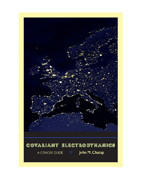Back to Results# Covariant Electrodynamics

A Concise Guide

John M. Charap

Publication Date

A notoriously difficult subject, covariant electrodynamics is nonetheless vital for understanding relativistic field theory. John M. Charap’s classroom-tested introduction to the mathematical foundations of the topic presents the material in an approachable manner.

Charap begins with a historical overview of electrodynamics and a discussion of the preliminary mathematics one needs in order to grasp the advanced and abstract concepts underlying the theory. He walks the reader through Maxwell’s four equations, explaining how they were developed and demonstrating how they are applied. From there...

A notoriously difficult subject, covariant electrodynamics is nonetheless vital for understanding relativistic field theory. John M. Charap’s classroom-tested introduction to the mathematical foundations of the topic presents the material in an approachable manner.

Charap begins with a historical overview of electrodynamics and a discussion of the preliminary mathematics one needs in order to grasp the advanced and abstract concepts underlying the theory. He walks the reader through Maxwell’s four equations, explaining how they were developed and demonstrating how they are applied. From there, Charap moves through the other components of electrodynamics, such as Lorentz transformations, tensors, and charged particle behavior. At each point, he carefully works through the mathematics, applies the concepts to simple physical systems, and provides historical context that makes clear the connections among the theories and the mathematicians responsible for developing them. A concluding chapter reviews the history of electrodynamics and points the way for independent testing of the theory.

Thorough, evenly paced, and intuitive, this friendly introduction to high-level covariant electrodynamics is a handy and helpful addition to any physicist’s toolkit.

Reviews

## Reviews

Like any good textbook that is intended for the purpose of teaching a course, there are numerous problems at the end of each chapter of varying degrees of difficulty.

John Charap succeeds well in making electrodynamics manifestly covariant, providing historical background and applications of far-reaching importance. The diligent reader, armed with pen and ample scratch paper for filling in the intermediate steps, will see covariant electrodynamics emerge coherently.

## Book Details

Publication Date
Status
Available
Trim Size
6.125
x
9.25
Pages
184
ISBN
9781421400150
Illustration Description
3 halftones, 9 line drawings
Subject

Preface
1. Introduction
2. Mathematical Preliminaries
2.1. A Reminder of Vector Calculus
2.2. Special Relativity
2.3. Four-Vectors
2.4. Covariant and Contravariant Vectors
2.5. Tensors
2.6. Time Dilation and

Preface
1. Introduction
2. Mathematical Preliminaries
2.1. A Reminder of Vector Calculus
2.2. Special Relativity
2.3. Four-Vectors
2.4. Covariant and Contravariant Vectors
2.5. Tensors
2.6. Time Dilation and the Lorentz-FitzGerald Contraction
2.7. The Four-Velocity
2.8. Energy and Momentum
2.9. Plane Waves
2.10. Exercises for Chapter 2
3. Maxwell's Equations
3.1. Our Starting Point
3.2. The Experimental Background
3.2.1. Coulomb's Law
3.2.2. Absence of Magnetic Monopoles
3.2.3. Ørsted and Ampere
3.2.4. The Law of Biot and Savart
3.2.5. The Displacement Current
3.2.7. The Lorentz Force
3.3. Capacitors and Solenoids
3.3.1. Energy
3.4. Electromagnetic Waves
3.4.1. Polarization
3.4.2. Electromagnetism and Light
3.5. Exercises for Chapter 3
4. Behavior under Lorentz Transformations
4.1. The Charge-Current Density Four-Vector
4.2. The Lorentz Force
4.3. The Potential Four-Vector
4.4. Gauge Transformations
4.5. The Field-Strength Tensor
4.6. The Dual Field-Strength Tensor
4.7. Exercises for Chapter 4
5. Lagrangian and Hamiltonian
5.1. Lagrange's Equations
5.2. The Lagrangian for a Charged Particle
5.3. The Hamiltonian for a Charged Particle
5.4. The Lagrangian for the Electromagnetic Field
5.5. The Hamiltonian for the Electromagnetic Field
5.6. Noether's Theorem
5.7. Exercises for Chapter 5
6. Stress, Energy, and Momentum
6.1. The Canonical Stress Tensor
6.2. The Symmetrical Stress Tensor
6.3. The Conservation Laws with Sources
6.4. The Field as an Ensemble of Oscillators
6.5. Exercises for Chapter 6
7. Motion of a Charged Particle
7.1. Fields from an Unaccelerated Particle
7.2. Motion of a Particle in an External Field
7.2.1. Uniform Static Magnetic Field
7.2.2. Crossed E and B Fields
7.2.3. Nonuniform Static B-Field
7.2.4. Curved Magnetic Field Lines
7.3. Exercises for Chapter 7
8. Fields from Sources
8.1. Introducing the Green's Function
8.2. The Delta Function
8.3. The Green's Function
8.4. The Covariant Form for the Green's Function
8.5. Exercises for Chapter 8
9.1. Potentials from a Moving Charged Particle
9.2. The Lienard-Wiechert Potentials
9.2.1. Fields from an Unaccelerated Particle
9.2.2. Fields from a Charged Oscillator
9.3. The General Case
9.4. The Multipole Expansion
9.4.2. Magnetic Dipole and Higher-Order Terms
9.5. Motion in a Circle
9.8. Exercises for Chapter 9
10. Media
10.1. Dispersion
10.1.1. Newton on the "Phænomena of Colours"
10.2. Refraction
10.2.1. The Boundary Conditions at the Interface
10.4. Exercises for Chapter 10
11. Scattering
11.1. Scattering from a Small Scatterer
11.2. Many Scatterers
11.3. Scattering from the Sky
11.3.1. The Born Approximation
11.3.2. Rayleigh's Explanation for the Blue Sky
11.4. Critical Opalescence
12. Dispersion
12.1. The Oscillator Model
12.1.1. The High-Frequency Limit
12.1.2. The Drude Model
12.2. Dispersion Relations
12.3. The Optical Theorem
Epilogue
Index

Author Bio
Featured Contributor

## John M. Charap

John M. Charap is an emeritus professor of theoretical physics at the University of London's Queen Mary College. He is the editor of Geometry of Constrained Dynamical Systems and the author of Explaining the Universe: The New Age of Physics.
Resources